# Bihar Board Class 10 Science Solutions Chapter 13 Magnetic Effects of Electric Current

BSEB Bihar Board Class 10 Science Solutions Chapter 13 Magnetic Effects of Electric Current Textbook Questions and Answers.

## Bihar Board Class 10 Science Solutions Chapter 13 Magnetic Effects of Electric Current

### Bihar Board Class 10 Science Chapter 13 Magnetic Effects of Electric Current InText Questions and Answers

In-text Questions (Page 224)

Question 1.
Why does a compass needle get deflected when brought near a bar magnet?
Magnetic compass needle and bar magnet both have magnetic field. When they are brought near each other, these magnetic fields interact with each other showing deflection in the needle.

In-text Questions (Page 228)

Question 1.
Draw magnetic field lines around a bar magnet.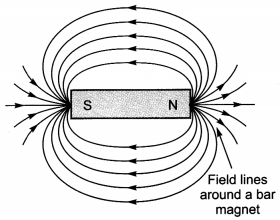Question 2.
List the properties of magnetic lines of force.
Properties of magnetic lines of force are listed below:

• Outside the magnet, the magnetic field lines are directed from N-pole of magnet towards S-pole. However, inside a magnet the field lines are directed from S-pole to N-pole. Thus magnetic field lines from a closed loop.
• The magnetic field line at any point, points in the direction of magnetic field at that point.
• The relative strength of magnetic fields is given by degree of closeness of the field lines. The magnetic field is strong in the region where the field lines are crowded.
• No two magnetic field lines can ever intersect with each other.

Question 3.
Why don’t two magnetic lines of force intersect each other?
Magnetic field-lines do not cross each other. If they did, it would mean that at the point of intersection, the compass needle would point towards two directions, which is not possible.

In-text Questions (Page 229-230)

Question 1.
Consider a circular loop of wire lying in the plane of the table. Let the current pass through the loop clockwise. Apply the right-hand rule to find out the direction of the magnetic field inside and outside the loop.
The magnetic field lines have been shown in figure given below. As per right-hand rule, we find that inside the loop, the magnetic field lines are directed perpendicular to the plane of paper in the inward direction. Outside the loop, magnetic field lines are directed out of the plane of paper.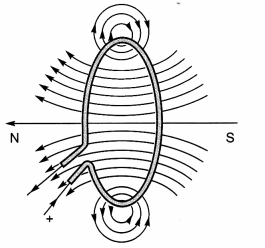Magnetic field lines of the field produced by a current carrying circular loop

Question 2.
The magnetic field in a given region is uniform. Draw a diagram to represent it.
The uniform magnetic field is represented by parallel, equidistant lines of equal length as shown in figure.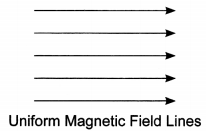Question 3.
Choose the correct option. The magnetic field inside a long straight solenoid-carrying current (a) is zero. (b) decreases as we move Uniform Magnetic Field Lines towards its end. (c) increases as we move towards its end. (d) is the same at all points.
(d) is the same at all points.

In-text Questions (Page 231-232)

Question 1.
Which of the following property of a proton can change while it moves freely in a magnetic field? (There may be more than one correct answer.) (a) Mass (6) Speed (c) Velocity (d) Momentum.
(c) Velocity and (d) Momentum.

Question 2.
In Activity 13.7, how do we think the displacement of rod AB will be affected if (i) current in rod AB is increased; (ii) a stronger horse-shoe magnet is used; and (iii) length of the rod AB is increased?
(i) If current in rod AB is increased, the displacement will also increase, (ii) If we use a stronger horse-shoe magnet then the displacement of rod AB will increase, (iii) If length of the rod is increased, force acting on it will increase and, hence, displacement of the rod will increase.

Question 3.
A positively-charged particle (alpha-particle) projected towards west is deflected towards north by a magnetic field. The direction of magnetic field is (a) towards south (b) towards east (c) downward (d) upward.
(d) upward in accordance with Fleming’s left-hand rule, the direction of magnetic field is vertically upward.

In-text Questions (Page 233)

Question 1.
State Fleming’s left-hand rule.
According to Fleming’s left-hand rule, stretch the thumb, forefinger and middle finger of your left hand such that they are mutually perpendicular. If the first finger points in the direction of magnetic field and the second finger in the direction of current, then the thumb will point in the direction of motion or the force acting on the conductor.

Question 2.
What is the principle of an electric motor?
The working principle of an electric motor is based on the magnetic effect of current. A current-carrying loop experiences a force and rotates when placed in a magnetic field. The direction of rotation of the loop is given by the Fleming’s left-hand rule.

Question 3.
What is the role of the split ring in an electric motor?
The split ring in the electric motor acts as a commutator. The commutator reverses the direction of current flowing through the coil after each half rotation of the coil. Due to this reversal of the current, the coil continues to rotate in the same direction.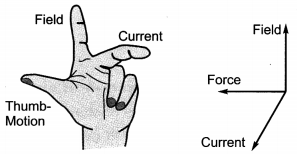In-text Questions (Page 236)

Question 1.
Explain different ways to induce current in a coil.
Different ways to induce current in a coil are as given below:

• If a magnetic field is changed around a coil then an induced current is set up in the coil. It can be done by taking a bar magnet and bringing it closer to the coil or taking it away from the coil.
• If a coil is moved in a magnetic field, then again an induced current is set up in the coil.
• If a coil is rotated in a uniform magnetic field, it may also produce an induced current in the coil.

In-text Questions (Page 237)

Question 1.
State the principle of an electric generator.
An electric generator is based on the principle of electromagnetic induction. When a rectangular coil is rotated in a uniform magnetic field, an induced voltage is generated between the ends of the coil.

Question 2.
Name some sources of direct current.
Some sources of direct current are a cell, a battery and a D.C. generator.

Question 3.
Which sources produce alternating current?
A.C. generator and invertors (used in house for emergency power supply) produces alternating current.

Question 4.
Choose the correct option.
A rectangular coil of copper wires is rotated in a magnetic field. The direction of the induced current changes once in each
(a) two revolutions
(b) one revolution
(c) half revolution
(d) one-fourth revolution
(c) When a rectangular coil of copper wire is rotated in a magnetic field, the direction of the induced current changes once in each half revolution.

In-text Questions (Page Page 238)

Question 1.
Name two safety measures commonly used in electric circuits and appliances.
Two safety measures are:

1. Use of earth wire and proper earthing.
2. Use of fuse (now-a-days fuse wire is replaced by MCB).

Question 2.
An electric oven of 2 kW power rating is operated in a domestic electric circuit (220 V) that has a current rating of 5 A. What result do you expect? Explain.
Power rating of electric oven P = 2 kW = 2000 W Supply voltage V = 220 V
So, the current drawn by the electric oven I = P/V = 2000 W/220 V = 9A

As the current rating of domestic electric circuit is only 5 A and the oven draws a current 9 A, which is more than the current rating, hence the circuit will be damaged due to overheating/overloading.

Question 3.
What precaution should be taken to avoid the overloading of domestic electric circuits?
The precautions that should be taken to avoid the overloading of domestic circuits are as follows:

• Too many appliances should not be connected to a single socket.
• Too many appliances should not be used at the same time.
• Faulty appliances should not be connected in the circuit.
• Fuse should be connected in the circuit.

### Bihar Board Class 10 Science Chapter 13 Magnetic Effects of Electric Current Textbook Questions and Answers

Question 1.
Which of the following correctly describes the magnetic field near a long straight wire?
(a) The field consists of straight lines perpendicular to the wire.
(b) The field consists of straight lines parallel to the wire.
(c) The field consists of radial lines originating from the wire.
(d) The field consists of concentric circles centred on the wire.
(d) The field consists of concentric circles centred on the wire.

Question 2.
The phenomenon of electromagnetic induction is
(а) the process of charging a body.
(b) the process of generating magnetic field due to a current passing through a coil.
(c) producing induced current in a coil due to relative motion between a magnet and the coil.
(d) the process of rotating a coil of an electric motor.
(c) producing induced current in a coil due to relative motion between a magnet and the coil.

Question 3.
The device used for producing electric current is called a
(a) generator
(b) galvanometer
(c) ammeter
(d) motor.
(a) generator

Question 4.
The essential difference between an AC generator and a DC generator is that
(a) AC generator has an electromagnet while a DC generator has permanent magnet
(b) DC generator will generate a higher voltage
(c) AC generator will generate a higher voltage
(d) AC generator has slip rings while the DC generator has a commutator.
(d) AC generator has slip rings while the DC generator has a commutator.

Question 5.
At the time of short circuit, the current in the circuit
(a) reduces substantially
(b) does not change
(c) increases heavily
(d) vary continuously.
(c) increases heavily

Question 6.
State whether the following statements are true or false.
(а) An electric motor converts mechanical energy into electrical energy.
(b) An electric generator works on the principle of electromagnetic induction.
(c) The field at the centre of a long circular coil carrying current will be parallel straight lines.
(d) A wire with a green insulation is usually the live wire of an electric supply.
(a) False
(b) True
(c) True
(d) False.

Question 7.
List two methods of producing magnetic fields.

1. Passing electric current through a straight conductor
2. Passing electric current through a circular loop
3. Passing electric current through a solenoid

Question 8.
How does a solenoid behave like a magnet? Can you determine the north and south poles of a current-carrying solenoid with the help of a bar magnet? Explain.
The pattern of the magnetic field around a current-carrying solenoid is same as that of a bar magnet. Yes, we can determine the poles of a solenoid by placing a bar magnet near it.

If north pole is placed near side B and repulsion is felt them B is North pole and vice versa.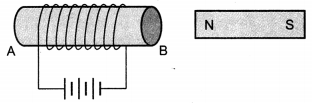Question 9.
When is the force experienced by a current-carrying conductor placed in a magnetic field largest?
When the direction of current is perpendicular to the direction of magnetic field in a current-carrying conductor.

Question 10.
Imagine that you are sitting in a chamber with your back to one wall. An electron beam, moving horizontally from back wall towards the front wall, is deflected by a strong magnetic field to your right side. What is the direction of magnetic field?
The direction of current (I) is opposite to the direction of the electron beam. Since the electron beam is deflected to the right side, applying, Fleming’s left hand rule, it is found that the direction of the magnetic field is vertically downwards.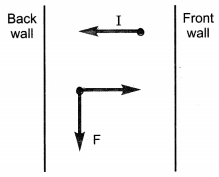Question 11.
Draw a labelled diagram of an electric motor. Explain its principle and working. What is the function of a spilt ring in an electric motor?
An electric motor is a rotating device that converts electrical energy to mechanical energy.
Principle: It works on the principle that when electric current is passed through a conductor placed perpendicularly in a magnetic field, a force acts on it as a result of which the conductor begins to move. An electrical motor, is shown in the figure given on the next page.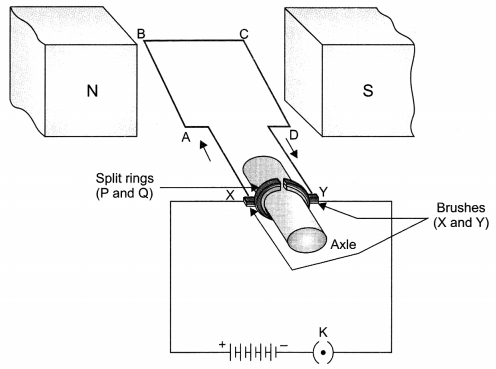Current in the coil ABCD enters from the source battery through conducting brush X and flows back to the battery through brush Y. The current in the arm AB of the coil flows from A to B. In arm CD it flows from C to D, that is, opposite to that through arm AB. On applying Fleming’s left hand rule for the direction of force on a current-carrying conductor in a magnetic field, we find that the force acting on arm AB pushes it downwards while the force acting on arm CD pushes it upwards. Thus the coil and the axle O rotate anti-clockwise. At half rotation, Q makes contact with the brush X and P with brush Y. Therefore the current in the coil gets reversed and flows along the path DCBA. The reversal of current also reverses the direction of force acting on the two arms AB and CD. Thus the arm AB of the coil that was earlier pushed down is now pushed up and the arm CD previously pushed up is now pushed down. Therefore the coil and the axle rotate half a turn more in the same direction. The reversing of the current is repeated at each half rotation, giving rise to a continuous rotation of the coil and to the axle.
The split ring reverses the direction of flow of current in an electric motor.

Question 12.
Name some devices in which electric motors are used.
Electric fans, refrigerators, mixers, washing machines, computers and CD players.

Question 13.
A coil of insulated copper wire is connected to a galvanometer. What will happen if a bar magnet is (i) pushed into the coil, (ii) withdrawn from inside the coil, (iii) held stationary inside the coil?
(i) The needle of the galvanometers moves momentarily in one direction
(ii) The needle of the galvanometers moves momentarily but in the opposite direction to (i)
(iii) No deflection of needle.

Question 14.
Two circular coils A and B are placed closed to each other. If the current in the coil A is changed, will some current be induced in the coil B? Give reason.
By changing current in the coil A, a current will be induced in coil B. This is because magnetic field associated with A changes when current in coil A is changed. This changes the magnetic field lines associated with coil B and thus a current is induced in it.

Question 15.
State the rule to determine the direction of a (i) magnetic field produced around a straight conductor-carrying current, (ii) force experienced by a current-carrying straight conductor placed in a magnetic field which is perpendicular to it, and (iii) current induced in a coil due to its rotation in a magnetic field.
(i) Imagine that you are holding a current-carrying straight conductor in your right hand such that thumb points towards the direction of current, then your fingers will wrap around the conductor in the direction of the field lines of the magnetic field, as shown in the figure. This is known as the right hand thumb rule.

(ii) Fleming’s left-hand rule determines the force on a conductor: According to this rule, stretch the thumb, forefinger and middle finger of your left hand such that they are mutually perpendicular.

If the fore-finger points in the direction of the magnetic field and the middle-finger in the direction of current, then the thumb will point in the direction of motion or force acting on the conductor.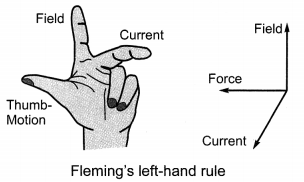(iii) Fleming’s right hand rule determines the direction of the induced current: Stretch the thumb, forefinger and middle finger of the right hand so that they are perpendicular to each other, as shown in figure. If the forefinger indicates the direction of the magnetic field and the thumb shows the direction of motion of the conductor, then the middle finger will show the direction of induced current. This simple rule is called Fleming’s right-hand rule.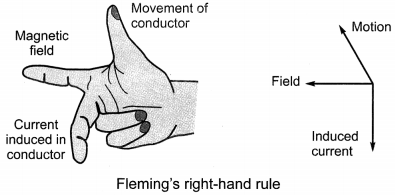Question 16.
Explain the underlying principle and working of an electric generator by drawing a labelled diagram. What is the function of brushes?
In an electric generator, mechanical energy is used to rotate a conductor in a magnetic field to produce electricity.
Principle: It works on the principle that when magnetic lines of force linked with a coil changes, a current is induced in the coil.

An electric generator is shown in the figure below.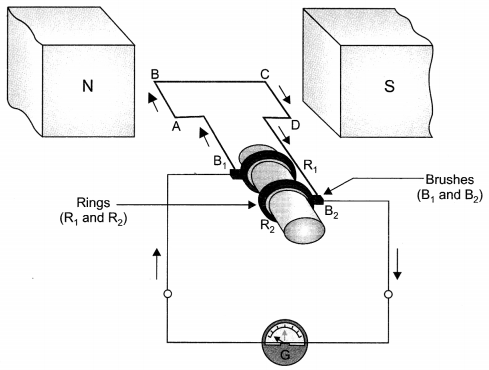When the coil ABCD is rotated clockwise, by applying Fleming’s right-hand rule, induced currents are set up in the these arms along the directions AB and CD. Thus an induced current flows in the direction ABCD. If there are larger number of turns in the coil, the current generated in each turn adds up to give a large current through the coil.

After half a rotation, arm CD starts moving up and AB moving down. As a result, the direction of the induced currents in both the arms change, giving rise to the net induced current in the direction DCBA. The current in the external circuit now flows from B1 and B2. Thus after every half rotation the polarity of the currnet in the respective arms changes. Such a current, which changes direction after equal intervals of time, is called an alternating current (abbreviated as AC). This device is called an AC generator.

The function of the brushes is to make contact with the rotating rings and through them supply current to the external circuit.

Question 17.
When does an electric short circuit occur?
Electric short-circuit occurs when live wire comes in direct contact with the neutral wire due to some fault in an appliance or damaged insulation of the wires.

Question 18.
What is the function of an earth wire? Why is it necessary to earth metallic appliances?
It is used as a safety measure to ensure that any leakage of current to a metallic body does not give severe shock to the user.

Earth wire provides a low resistance conducting path for electric current to the earth which may have leaked from an appliance.

### Bihar Board Class 10 Science Chapter 13 Magnetic Effects of Electric Current Textbook Activities

Activity 13.1 (NCERT Textbook Page 223)

Discussion and conclusion:
The needle of the compass is deflected when electric current is passed through it since electric current produces a magnetic field.

Activity 13.2 (NCERT Textbook Page 224)

Discussion and conclusion:
The iron filings arrange themselves in a pattern. The magnet exerts its influence in the region surrounding it. This region surrounding the magnet is called magnetic field.

Activity 13.4 (NCERT Textbook Page 226)

Discussion and conclusion:
The direction of magnetic field due to a current carrying straight conductor depends upon the direction of current flowing through it. If we reverse the direction of current the direction of magnetic field is also reversed.

Activity 13.7 (NCERT Textbook Page 230)

Discussion and conclusion:
Current carrying wire placed in magnetic field experience force. On reversing the direction of current, the direction of force is reversed.

Activity 13.8 (NCERT Textbook Page 233-234)

Discussion and conclusion:

• An emf and current is induced in the circuit when magnetic field linked with the coil changes.
• When the magnet is stationary, magnetic field linked with the coil does not change. Hence there is no induced emf and current in the coil.
• The direction of induced current depends on the direction of motion of the magnet.

Activity 13.9 (NCERT Textbook Page 235)

Discussion and conclusion:

• Whenever electric current through coil-1 is changed (either increased or decreased), a pd is induced in coil-2.
• The coil-1 (containing battery) is called the primary coil whereas coil-2 is called the secondary coil.
• The induced pd in coil-2 is due to change in magnetic field lines in it which in turn is due to change in magnetic field lines in coil-1 through which current is changed.